Problem
Suppose that an inflating balloon is spherical in shape, and its radius is changing at the rate of 3 centimeters per second. At what rate is the volume changing when the radius is 10 centimeters?
Solution
The relation between the radius (r) and the volume (V) of a sphere is given by the formula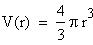Since the volume and radius are both dependent on time (so r and V are functions of t), when we differentiate the above equation we use the chain rule, to get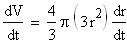Substituting what we know,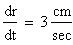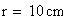we get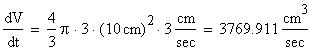So the volume of the balloon is increasing by about 3770 cubic centimeters per second when the radius is 10 centimeters.